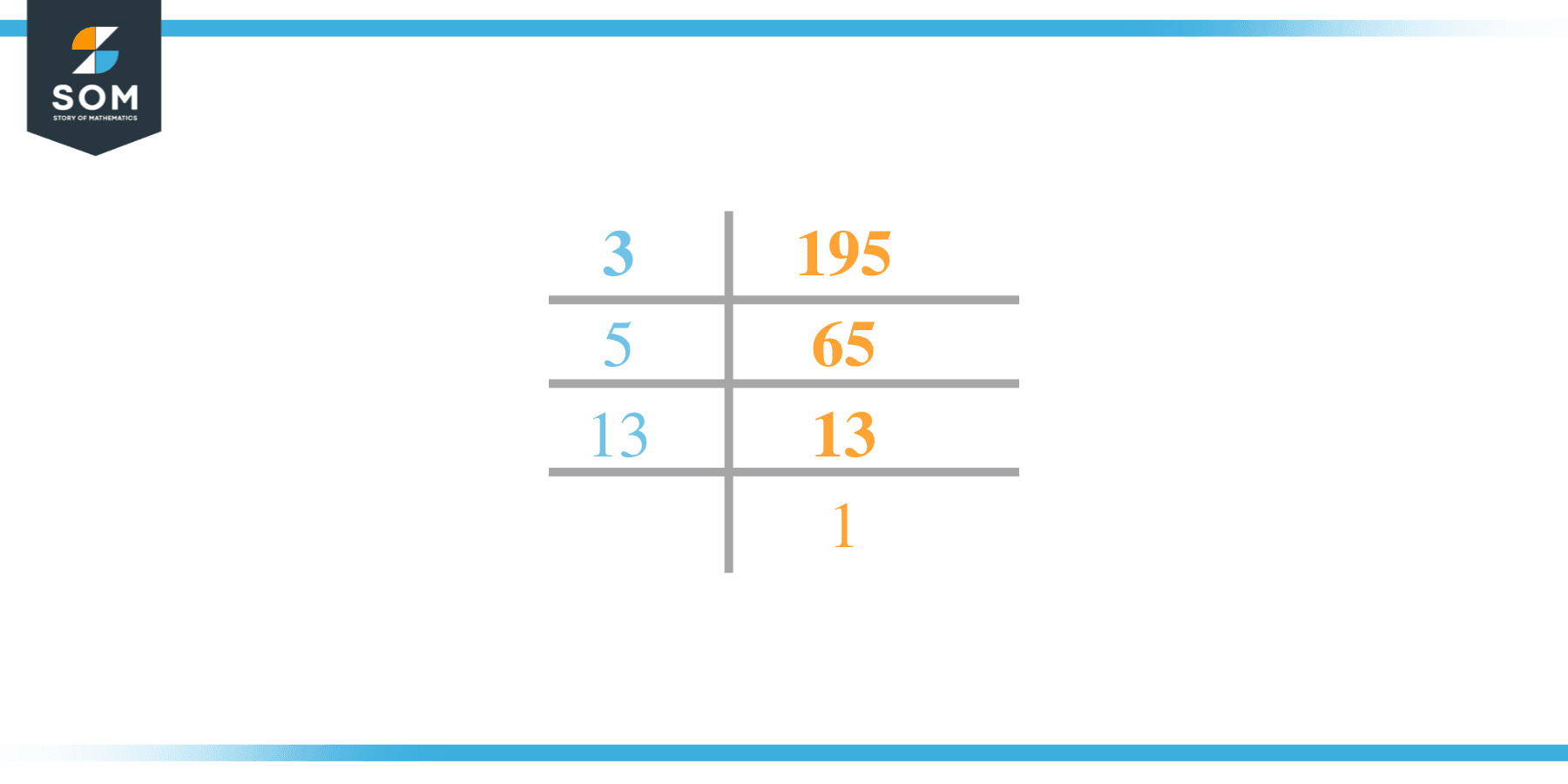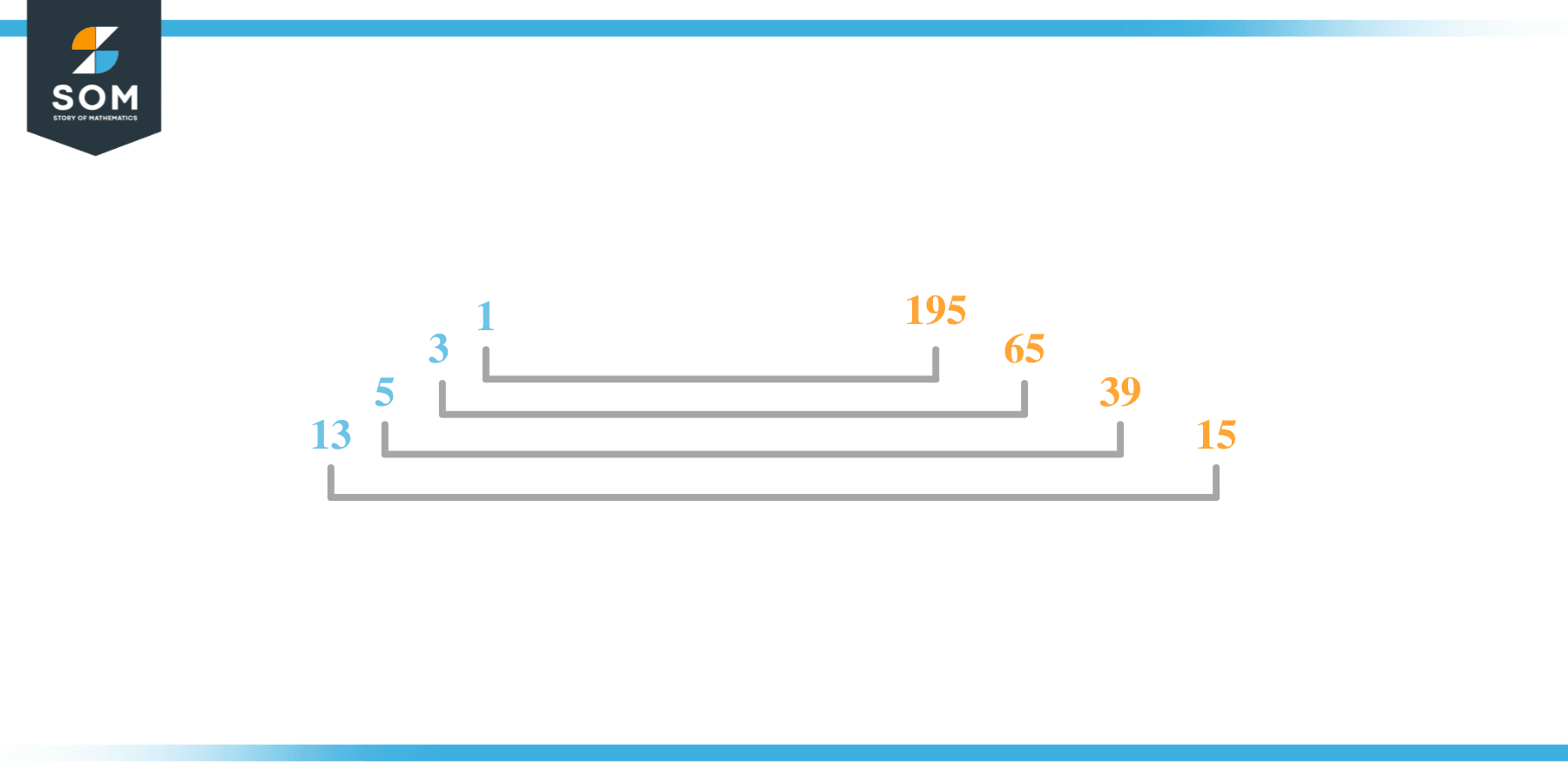# Factors of 195: Prime Factorization, Methods, and Examples

The factors of 195 are the numbers that give zero as the remainder whenever 195 is divided from them. These factors can be both positive and negative and they also form factor pairs with one another.The number 195 is an odd composite number.

### Factors of 195

Here are the factors of number 195.

Factors of 195: 1, 3, 5, 13, 15, 39, 65, 195

### Negative Factors of 195

The negative factors of 195 are similar to its positive factors, just with a negative sign.

Negative Factors of 195: -1, -3, -5, -13, -15, -39, -65 and -195

### Prime Factorization of 195

The prime factorization of 195 is the way of expressing its prime factors in the product form.

Prime Factorization: 3 x 5 x 13

In this article, we will learn about the factors of 195 and how to find them using various techniques such as upside-down division, prime factorization, and factor tree.

## What Are the Factors of 195?

The factors of 195 are 1, 3, 5, 13, 15, 39, 65, and 195. All of these numbers are the factors as they do not leave any remainder when divided by 195.

The factors of 195 are classified as prime numbers and composite numbers. The prime factors of the number 195 can be determined using the technique of prime factorization.

## How To Find the Factors of 195?

You can find the factors of 195 by using the rules of divisibility. The divisibility rule states that any number, when divided by any other natural number, is said to be divisible by the number if the quotient is the whole number and the resulting remainder is zero.

To find the factors of 195, create a list containing the numbers that are exactly divisible by 195 with zero remainders. One important thing to note is that 1 and 195 are the 195’s factors as every natural number has 1 and the number itself as its factor.

1 is also called the universal factor of every number. The factors of 195 are determined as follows:

$\dfrac{195}{1} = 195$

$\dfrac{195}{3} = 65$

$\dfrac{195}{5} = 39$

$\dfrac{195}{13} = 15$

$\dfrac{195}{15} =13$

$\dfrac{195}{39}= 5$

$\dfrac{195}{65} =3$

$\dfrac{195}{195}=1$

Therefore, 1, 3, 5, 13, 15, 39, 65, and 195 are the factors of 195.

### Total Number of Factors of 195

For 195 there are 8 positive factors and 8 negative ones. So in total, there are 16 factors of 195.

To find the total number of factors of the given number, follow the procedure mentioned below:

1. Find the factorization of the given number.
2. Demonstrate the prime factorization of the number in the form of exponent form.
3. Add 1 to each of the exponents of the prime factor.
4. Now, multiply the resulting exponents together. This obtained product is equivalent to the total number of factors of the given number.

By following this procedure the total number of factors of 195 is given as:

Factorization of 195 is 1 x 3 x 5 x 13

The exponent of all factors is 1.

Adding 1 to each and multiplying them together results in 16.

Therefore, the total number of factors of 195 is 16, where 8 are positive factors and 8 are negative.

### Important Notes

Here are some important points that must be considered while finding the factors of any given number:

• The factor of any given number must be a whole number.
• The factors of the number cannot be in the form of decimals or fractions.
• Factors can be positive as well as negative.
• Negative factors are the additive inverse of the positive factors of a given number.
• The factor of a number cannot be greater than that number.
• Every even number has 2 as its prime factor, the smallest prime factor.

## Factors of 195 by Prime FactorizationThe number 195 is a composite number. Prime factorization is a useful technique for finding the number’s prime factors and expressing the number as the product of its prime factors.

Before finding the factors of 195 using prime factorization, let us find out what prime factors are. Prime factors are the factors of any given number that are only divisible by 1 and themselves.

To start the prime factorization of 195, start dividing by its smallest prime factor. First, determine that the given number is either even or odd. If it is an even number, then 2 will be the smallest prime factor.

Continue splitting the quotient obtained until 1 is received as the quotient. The prime factorization of 195 can be expressed as:

195 = 3 x 5 x 13

## Factors of 195 in PairsThe factor pairs are the duplet of numbers that when multiplied together result in the factorized number. Factor pairs can be more than one depending on the total number of factors of the given numbers.

For 195, the factor pairs can be found as:

1 x 195 = 195

3 x 65 = 195

5 x 39 =195

13 x 15 = 195

The possible factor pairs of 195 are given as (1, 195), (3, 65), (5, 39), and (13, 15).

All these numbers in pairs, when multiplied, give 195 as the product.

The negative factor pairs of 195 are given as:

-1 x -195 = 195

-3 x -65 = 195

-5 x -39 =195

-13 x -15 = 195

It is important to note that in negative factor pairs, the minus sign has been multiplied by the minus sign due to which the resulting product is the original positive number. Therefore, -1, -3, -5, -13, -15, -39, -65, and -195 are called negative factors of 195.

The list of all the factors of 195 including positive as well as negative numbers is given below.

Factor list of 195: 1, -1, 3, -3, 5, -5, 13, -13, 15, -15, 39, -39, 65, -65, 195, and -195

## Factors of 195 Solved Examples

To better understand the concept of factors, let’s solve some examples.

### Example 1

How many factors of 195 are there?

### Solution

The total number of Factors of 195 is 8.

Factors of 195 are 1, 3, 5, 13, 15, 39, 65, and 195.

### Example 2

Find the factors of 195 using prime factorization.

### Solution

The prime factorization of 195 is given as:

195 $\div$ 5 = 39

39 $\div$ 3 = 13

13 $\div$ 13 =1

So the prime factorization of 195 can be written as:

3 x 5 x 13 = 195